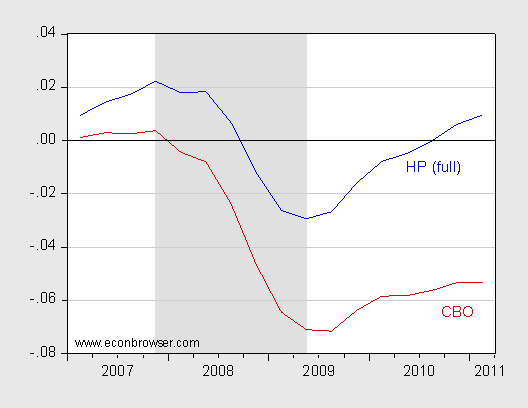# Measure the affinity between two functions

I just want to know, what is the process of measure the affinity of two functions. I have no idea about if it has a proper name at all, so bear with me.

For example, given two functions f(x) and g(x), I would like to know how "similar" they are in the same domain. I am not talking about values, but how they vary on similar values of the domain.

For example. given f(x) = cycle speed and g(x) = temperature in degrees, using the same time span as domain a day during winter, probably when the temperature starts to get high in the noon, also the speed of the cyclist. Then if you represent both graphs, visually you can see the relation, now I would like to measure that relationship mathematically. I would like to get many functions that represents different elements, and measure how similar they are to the cyclist speed graph in terms of variation.

For example:Forget the scale in the Y axis; function blue and red seems to have some likeness, even if they consider different magnitudes, values, and even some offset in the variations... they seem to "move close each other". Blue may be the cyclist speed in m/s, and red the temperature in degrees, but despite of different magnitudes... it is obvious that there is some kind of relationship, where one influences the other.

Even calculating the derivative of each, they may be different because the speed of the cyclist does not change as soon as the temperature change, it takes a while, so there maybe some offset.

I would like to know the name of that relationship, and the name of the techniques to calculate it.

What you are looking for sounds pretty much like the cross-correlation, which in turn is an extension of the correlation. The general ideas are these:

The correlation between two functions is a measure for their similarity without taking delays into account. Different correlation coefficients have been suggested in the past and which one is most appropriate somewhat depends on what you know or assume about your data. The most appropriate one for your problem is probably Pearson’s linear correlation coefficient $r_{f,g}$, which measures, roughly speaking, how well you can overlap the graphs of $f$ and $g$ by vertical movement and stretching. The restriction to vertical movements here means that delays are not taken into account.

The cross-correlation is nothing but the correlation with one function’s graph being horizontally shifted by some delay $τ$:

$$ρ_{f,g}(τ) := r_{f,\tilde{g}_τ} \qquad \text{with } \tilde{g}_τ(t)=g(t+τ)$$

If $ρ_{f,g}$ is maximal for some $τ$, this is your optimal overlap and the corresponding cross-correlation quantifies the similarity between your functions, or if you so wish: the affinity of their graphs without considering horizontal stretching.

While the concept of cross-correlation is usually applied to the linear correlation coefficient, it’s easy to make analogues for other correlation coefficients.

One sidenote: If you want to analyse real and thus discrete data and not actual functions, you are entering the realm of time-series analysis.

• Thanks, it looks pretty much what I was looking for. Dec 2, 2014 at 13:08